# Pointers

In earlier chapters, variables have been explained as locations in the computer's memory which can be accessed by their identifier (their name). This way, the program does not need to care about the physical address of the data in memory; it simply uses the identifier whenever it needs to refer to the variable.

For a C++ program, the memory of a computer is like a succession of memory cells, each one byte in size, and each with a unique address. These single-byte memory cells are ordered in a way that allows data representations larger than one byte to occupy memory cells that have consecutive addresses.

This way, each cell can be easily located in the memory by means of its unique address. For example, the memory cell with the address `1776` always follows immediately after the cell with address `1775` and precedes the one with `1777`, and is exactly one thousand cells after `776` and exactly one thousand cells before `2776`.

When a variable is declared, the memory needed to store its value is assigned a specific location in memory (its memory address). Generally, C++ programs do not actively decide the exact memory addresses where its variables are stored. Fortunately, that task is left to the environment where the program is run - generally, an operating system that decides the particular memory locations on runtime. However, it may be useful for a program to be able to obtain the address of a variable during runtime in order to access data cells that are at a certain position relative to it.

The address of a variable can be obtained by preceding the name of a variable with an ampersand sign (`&`), known as address-of operator. For example:

 ``1`` ``foo = &myvar;``

This would assign the address of variable `myvar` to `foo`; by preceding the name of the variable `myvar` with the address-of operator (`&`), we are no longer assigning the content of the variable itself to `foo`, but its address.

The actual address of a variable in memory cannot be known before runtime, but let's assume, in order to help clarify some concepts, that `myvar` is placed during runtime in the memory address `1776`.

In this case, consider the following code fragment:

 ``123`` ``````myvar = 25; foo = &myvar; bar = myvar;``````

The values contained in each variable after the execution of this are shown in the following diagram: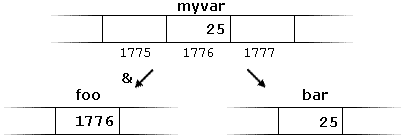First, we have assigned the value `25` to `myvar` (a variable whose address in memory we assumed to be `1776`).

The second statement assigns `foo` the address of `myvar`, which we have assumed to be `1776`.

Finally, the third statement, assigns the value contained in `myvar` to `bar`. This is a standard assignment operation, as already done many times in earlier chapters.

The main difference between the second and third statements is the appearance of the address-of operator (`&`).

The variable that stores the address of another variable (like `foo` in the previous example) is what in C++ is called a pointer. Pointers are a very powerful feature of the language that has many uses in lower level programming. A bit later, we will see how to declare and use pointers.

### Dereference operator (*)

As just seen, a variable which stores the address of another variable is called a pointer. Pointers are said to "point to" the variable whose address they store.

An interesting property of pointers is that they can be used to access the variable they point to directly. This is done by preceding the pointer name with the dereference operator (`*`). The operator itself can be read as "value pointed to by".

Therefore, following with the values of the previous example, the following statement:

 ``1`` ``baz = *foo;``

This could be read as: "`baz` equal to value pointed to by `foo`", and the statement would actually assign the value `25` to `baz`, since `foo` is `1776`, and the value pointed to by `1776` (following the example above) would be `25`.It is important to clearly differentiate that `foo` refers to the value `1776`, while `*foo` (with an asterisk `*` preceding the identifier) refers to the value stored at address `1776`, which in this case is `25`. Notice the difference of including or not including the dereference operator (I have added an explanatory comment of how each of these two expressions could be read):

 ``12`` ``````baz = foo; // baz equal to foo (1776) baz = *foo; // baz equal to value pointed to by foo (25) ``````

The reference and dereference operators are thus complementary:
• `&` is the address-of operator, and can be read simply as "address of"
• `*` is the dereference operator, and can be read as "value pointed to by"

Thus, they have sort of opposite meanings: An address obtained with `&` can be dereferenced with `*`.

Earlier, we performed the following two assignment operations:

 ``12`` ``````myvar = 25; foo = &myvar;``````

Right after these two statements, all of the following expressions would give true as result:

 ``1234`` ``````myvar == 25 &myvar == 1776 foo == 1776 *foo ==``````

The first expression is quite clear, considering that the assignment operation performed on `myvar` was `myvar=25`. The second one uses the address-of operator (`&`), which returns the address of `myvar`, which we assumed it to have a value of `1776`. The third one is somewhat obvious, since the second expression was true and the assignment operation performed on `foo` was `foo=&myvar`. The fourth expression uses the dereference operator (`*`) that can be read as "value pointed to by", and the value pointed to by `foo` is indeed `25`.

So, after all that, you may also infer that for as long as the address pointed to by `foo` remains unchanged, the following expression will also be true:

 ``1`` ``*foo ==``

### Declaring pointers

Due to the ability of a pointer to directly refer to the value that it points to, a pointer has different properties when it points to a `char` than when it points to an `int` or a `float`. Once dereferenced, the type needs to be known. And for that, the declaration of a pointer needs to include the data type the pointer is going to point to.

The declaration of pointers follows this syntax:

`type * name;`

where `type` is the data type pointed to by the pointer. This type is not the type of the pointer itself, but the type of the data the pointer points to. For example:

 ``123`` ``````int * number; char * character; double * decimals;``````

These are three declarations of pointers. Each one is intended to point to a different data type, but, in fact, all of them are pointers and all of them are likely going to occupy the same amount of space in memory (the size in memory of a pointer depends on the platform where the program runs). Nevertheless, the data to which they point to do not occupy the same amount of space nor are of the same type: the first one points to an `int`, the second one to a `char`, and the last one to a `double`. Therefore, although these three example variables are all of them pointers, they actually have different types: `int*`, `char*`, and `double*` respectively, depending on the type they point to.

Note that the asterisk (`*`) used when declaring a pointer only means that it is a pointer (it is part of its type compound specifier), and should not be confused with the dereference operator seen a bit earlier, but which is also written with an asterisk (`*`). They are simply two different things represented with the same sign.

Let's see an example on pointers:

 ``````// my first pointer #include using namespace std; int main () { int firstvalue, secondvalue; int * mypointer; mypointer = &firstvalue; *mypointer = 10; mypointer = &secondvalue; *mypointer = 20; cout << "firstvalue is " << firstvalue << '\n'; cout << "secondvalue is " << secondvalue << '\n'; return 0; }`````` ```firstvalue is 10 secondvalue is 20```

Notice that even though neither `firstvalue` nor `secondvalue` are directly set any value in the program, both end up with a value set indirectly through the use of `mypointer`. This is how it happens:

First, `mypointer` is assigned the address of firstvalue using the address-of operator (`&`). Then, the value pointed to by `mypointer` is assigned a value of `10`. Because, at this moment, `mypointer` is pointing to the memory location of `firstvalue`, this in fact modifies the value of `firstvalue`.

In order to demonstrate that a pointer may point to different variables during its lifetime in a program, the example repeats the process with `secondvalue` and that same pointer, `mypointer`.

Here is an example a little bit more elaborated:

 ``````// more pointers #include using namespace std; int main () { int firstvalue = 5, secondvalue = 15; int * p1, * p2; p1 = &firstvalue; // p1 = address of firstvalue p2 = &secondvalue; // p2 = address of secondvalue *p1 = 10; // value pointed to by p1 = 10 *p2 = *p1; // value pointed to by p2 = value pointed to by p1 p1 = p2; // p1 = p2 (value of pointer is copied) *p1 = 20; // value pointed to by p1 = 20 cout << "firstvalue is " << firstvalue << '\n'; cout << "secondvalue is " << secondvalue << '\n'; return 0; }`````` ```firstvalue is 10 secondvalue is 20```

Each assignment operation includes a comment on how each line could be read: i.e., replacing ampersands (`&`) by "address of", and asterisks (`*`) by "value pointed to by".

Notice that there are expressions with pointers `p1` and `p2`, both with and without the dereference operator (`*`). The meaning of an expression using the dereference operator (*) is very different from one that does not. When this operator precedes the pointer name, the expression refers to the value being pointed, while when a pointer name appears without this operator, it refers to the value of the pointer itself (i.e., the address of what the pointer is pointing to).

Another thing that may call your attention is the line:

 ``1`` ``int * p1, * p2;``

This declares the two pointers used in the previous example. But notice that there is an asterisk (`*`) for each pointer, in order for both to have type `int*` (pointer to `int`). This is required due to the precedence rules. Note that if, instead, the code was:

 ``1`` ``int * p1, p2;``

`p1` would indeed be of type `int*`, but `p2` would be of type `int`. Spaces do not matter at all for this purpose. But anyway, simply remembering to put one asterisk per pointer is enough for most pointer users interested in declaring multiple pointers per statement. Or even better: use a different statement for each variable.

### Pointers and arrays

The concept of arrays is related to that of pointers. In fact, arrays work very much like pointers to their first elements, and, actually, an array can always be implicitly converted to the pointer of the proper type. For example, consider these two declarations:

 ``12`` ``````int myarray ; int * mypointer;``````

The following assignment operation would be valid:

 ``1`` ``mypointer = myarray;``

After that, `mypointer` and `myarray` would be equivalent and would have very similar properties. The main difference being that `mypointer` can be assigned a different address, whereas `myarray` can never be assigned anything, and will always represent the same block of 20 elements of type `int`. Therefore, the following assignment would not be valid:

 ``1`` ``myarray = mypointer;``

Let's see an example that mixes arrays and pointers:

 ``````// more pointers #include using namespace std; int main () { int numbers; int * p; p = numbers; *p = 10; p++; *p = 20; p = &numbers; *p = 30; p = numbers + 3; *p = 40; p = numbers; *(p+4) = 50; for (int n=0; n<5; n++) cout << numbers[n] << ", "; return 0; }`````` `10, 20, 30, 40, 50, `

Pointers and arrays support the same set of operations, with the same meaning for both. The main difference being that pointers can be assigned new addresses, while arrays cannot.

In the chapter about arrays, brackets (`[]`) were explained as specifying the index of an element of the array. Well, in fact these brackets are a dereferencing operator known as offset operator. They dereference the variable they follow just as `*` does, but they also add the number between brackets to the address being dereferenced. For example:

 ``12`` ``````a = 0; // a [offset of 5] = 0 *(a+5) = 0; // pointed to by (a+5) = 0 ``````

These two expressions are equivalent and valid, not only if `a` is a pointer, but also if `a` is an array. Remember that if an array, its name can be used just like a pointer to its first element.

### Pointer initialization

Pointers can be initialized to point to specific locations at the very moment they are defined:

 ``12`` ``````int myvar; int * myptr = &myvar;``````

The resulting state of variables after this code is the same as after:

 ``123`` ``````int myvar; int * myptr; myptr = &myvar;``````

When pointers are initialized, what is initialized is the address they point to (i.e., `myptr`), never the value being pointed (i.e., `*myptr`). Therefore, the code above shall not be confused with:

 ``123`` ``````int myvar; int * myptr; *myptr = &myvar;``````

Which anyway would not make much sense (and is not valid code).

The asterisk (`*`) in the pointer declaration (line 2) only indicates that it is a pointer, it is not the dereference operator (as in line 3). Both things just happen to use the same sign: `*`. As always, spaces are not relevant, and never change the meaning of an expression.

Pointers can be initialized either to the address of a variable (such as in the case above), or to the value of another pointer (or array):

 ``123`` ``````int myvar; int *foo = &myvar; int *bar = foo;``````

### Pointer arithmetics

To conduct arithmetical operations on pointers is a little different than to conduct them on regular integer types. To begin with, only addition and subtraction operations are allowed; the others make no sense in the world of pointers. But both addition and subtraction have a slightly different behavior with pointers, according to the size of the data type to which they point.

When fundamental data types were introduced, we saw that types have different sizes. For example: `char` always has a size of 1 byte, `short` is generally larger than that, and `int` and `long` are even larger; the exact size of these being dependent on the system. For example, let's imagine that in a given system, `char` takes 1 byte, `short` takes 2 bytes, and `long` takes 4.

Suppose now that we define three pointers in this compiler:

 ``123`` ``````char *mychar; short *myshort; long *mylong;``````

and that we know that they point to the memory locations `1000`, `2000`, and `3000`, respectively.

Therefore, if we write:

 ``123`` ``````++mychar; ++myshort; ++mylong;``````

`mychar`, as one would expect, would contain the value 1001. But not so obviously, `myshort` would contain the value 2002, and `mylong` would contain 3004, even though they have each been incremented only once. The reason is that, when adding one to a pointer, the pointer is made to point to the following element of the same type, and, therefore, the size in bytes of the type it points to is added to the pointer.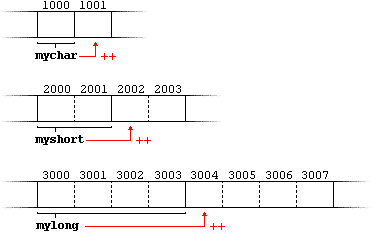This is applicable both when adding and subtracting any number to a pointer. It would happen exactly the same if we wrote:

 ``123`` ``````mychar = mychar + 1; myshort = myshort + 1; mylong = mylong + 1;``````

Regarding the increment (`++`) and decrement (`--`) operators, they both can be used as either prefix or suffix of an expression, with a slight difference in behavior: as a prefix, the increment happens before the expression is evaluated, and as a suffix, the increment happens after the expression is evaluated. This also applies to expressions incrementing and decrementing pointers, which can become part of more complicated expressions that also include dereference operators (`*`). Remembering operator precedence rules, we can recall that postfix operators, such as increment and decrement, have higher precedence than prefix operators, such as the dereference operator (`*`). Therefore, the following expression:

 ``1`` ``*p++``

is equivalent to `*(p++)`. And what it does is to increase the value of `p` (so it now points to the next element), but because `++` is used as postfix, the whole expression is evaluated as the value pointed originally by the pointer (the address it pointed to before being incremented).

Essentially, these are the four possible combinations of the dereference operator with both the prefix and suffix versions of the increment operator (the same being applicable also to the decrement operator):

 ``1234`` ``````*p++ // same as *(p++): increment pointer, and dereference unincremented address *++p // same as *(++p): increment pointer, and dereference incremented address ++*p // same as ++(*p): dereference pointer, and increment the value it points to (*p)++ // dereference pointer, and post-increment the value it points to ``````

A typical -but not so simple- statement involving these operators is:

 ``1`` ``*p++ = *q++;``

Because `++` has a higher precedence than `*`, both `p` and `q` are incremented, but because both increment operators (`++`) are used as postfix and not prefix, the value assigned to `*p` is `*q` before both `p` and `q` are incremented. And then both are incremented. It would be roughly equivalent to:

 ``123`` ``````*p = *q; ++p; ++q;``````

Like always, parentheses reduce confusion by adding legibility to expressions.

### Pointers and const

Pointers can be used to access a variable by its address, and this access may include modifying the value pointed. But it is also possible to declare pointers that can access the pointed value to read it, but not to modify it. For this, it is enough with qualifying the type pointed to by the pointer as `const`. For example:

 ``12345`` ``````int x; int y = 10; const int * p = &y; x = *p; // ok: reading p *p = x; // error: modifying p, which is const-qualified ``````

Here `p` points to a variable, but points to it in a `const`-qualified manner, meaning that it can read the value pointed, but it cannot modify it. Note also, that the expression `&y` is of type `int*`, but this is assigned to a pointer of type `const int*`. This is allowed: a pointer to non-const can be implicitly converted to a pointer to const. But not the other way around! As a safety feature, pointers to `const` are not implicitly convertible to pointers to non-`const`.

One of the use cases of pointers to `const` elements is as function parameters: a function that takes a pointer to non-`const` as parameter can modify the value passed as argument, while a function that takes a pointer to `const` as parameter cannot.

 ``````// pointers as arguments: #include using namespace std; void increment_all (int* start, int* stop) { int * current = start; while (current != stop) { ++(*current); // increment value pointed ++current; // increment pointer } } void print_all (const int* start, const int* stop) { const int * current = start; while (current != stop) { cout << *current << '\n'; ++current; // increment pointer } } int main () { int numbers[] = {10,20,30}; increment_all (numbers,numbers+3); print_all (numbers,numbers+3); return 0; }`````` ```11 21 31```

Note that `print_all` uses pointers that point to constant elements. These pointers point to constant content they cannot modify, but they are not constant themselves: i.e., the pointers can still be incremented or assigned different addresses, although they cannot modify the content they point to.

And this is where a second dimension to constness is added to pointers: Pointers can also be themselves const. And this is specified by appending const to the pointed type (after the asterisk):

 ``12345`` ``````int x; int * p1 = &x; // non-const pointer to non-const int const int * p2 = &x; // non-const pointer to const int int * const p3 = &x; // const pointer to non-const int const int * const p4 = &x; // const pointer to const int ``````

The syntax with `const` and pointers is definitely tricky, and recognizing the cases that best suit each use tends to require some experience. In any case, it is important to get constness with pointers (and references) right sooner rather than later, but you should not worry too much about grasping everything if this is the first time you are exposed to the mix of `const` and pointers. More use cases will show up in coming chapters.

To add a little bit more confusion to the syntax of `const` with pointers, the `const` qualifier can either precede or follow the pointed type, with the exact same meaning:

 ``12`` ``````const int * p2a = &x; // non-const pointer to const int int const * p2b = &x; // also non-const pointer to const int ``````

As with the spaces surrounding the asterisk, the order of const in this case is simply a matter of style. This chapter uses a prefix `const`, as for historical reasons this seems to be more extended, but both are exactly equivalent. The merits of each style are still intensely debated on the internet.

### Pointers and string literals

As pointed earlier, string literals are arrays containing null-terminated character sequences. In earlier sections, string literals have been used to be directly inserted into `cout`, to initialize strings and to initialize arrays of characters.

But they can also be accessed directly. String literals are arrays of the proper array type to contain all its characters plus the terminating null-character, with each of the elements being of type `const char` (as literals, they can never be modified). For example:

 ``1`` ``const char * foo = "hello";``

This declares an array with the literal representation for `"hello"`, and then a pointer to its first element is assigned to `foo`. If we imagine that `"hello"` is stored at the memory locations that start at address 1702, we can represent the previous declaration as:Note that here `foo` is a pointer and contains the value 1702, and not `'h'`, nor `"hello"`, although 1702 indeed is the address of both of these.

The pointer `foo` points to a sequence of characters. And because pointers and arrays behave essentially in the same way in expressions, `foo` can be used to access the characters in the same way arrays of null-terminated character sequences are. For example:

 ``12`` ``````*(foo+4) foo``````

Both expressions have a value of `'o'` (the fifth element of the array).

### Pointers to pointers

C++ allows the use of pointers that point to pointers, that these, in its turn, point to data (or even to other pointers). The syntax simply requires an asterisk (`*`) for each level of indirection in the declaration of the pointer:

 ``123456`` ``````char a; char * b; char ** c; a = 'z'; b = &a; c = &b;``````

This, assuming the randomly chosen memory locations for each variable of `7230`, `8092`, and `10502`, could be represented as: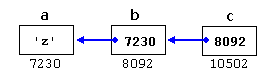With the value of each variable represented inside its corresponding cell, and their respective addresses in memory represented by the value under them.

The new thing in this example is variable `c`, which is a pointer to a pointer, and can be used in three different levels of indirection, each one of them would correspond to a different value:

• `c` is of type `char**` and a value of `8092`
• `*c` is of type `char*` and a value of `7230`
• `**c` is of type `char` and a value of `'z'`

### void pointers

The `void` type of pointer is a special type of pointer. In C++, `void` represents the absence of type. Therefore, `void` pointers are pointers that point to a value that has no type (and thus also an undetermined length and undetermined dereferencing properties).

This gives `void` pointers a great flexibility, by being able to point to any data type, from an integer value or a float to a string of characters. In exchange, they have a great limitation: the data pointed to by them cannot be directly dereferenced (which is logical, since we have no type to dereference to), and for that reason, any address in a `void` pointer needs to be transformed into some other pointer type that points to a concrete data type before being dereferenced.

One of its possible uses may be to pass generic parameters to a function. For example:

 ``````// increaser #include using namespace std; void increase (void* data, int psize) { if ( psize == sizeof(char) ) { char* pchar; pchar=(char*)data; ++(*pchar); } else if (psize == sizeof(int) ) { int* pint; pint=(int*)data; ++(*pint); } } int main () { char a = 'x'; int b = 1602; increase (&a,sizeof(a)); increase (&b,sizeof(b)); cout << a << ", " << b << '\n'; return 0; }`````` `y, 1603`

`sizeof` is an operator integrated in the C++ language that returns the size in bytes of its argument. For non-dynamic data types, this value is a constant. Therefore, for example, `sizeof(char)` is 1, because `char` has always a size of one byte.

### Invalid pointers and null pointers

In principle, pointers are meant to point to valid addresses, such as the address of a variable or the address of an element in an array. But pointers can actually point to any address, including addresses that do not refer to any valid element. Typical examples of this are uninitialized pointers and pointers to nonexistent elements of an array:

 ``1234`` ``````int * p; // uninitialized pointer (local variable) int myarray; int * q = myarray+20; // element out of bounds ``````

Neither `p` nor `q` point to addresses known to contain a value, but none of the above statements causes an error. In C++, pointers are allowed to take any address value, no matter whether there actually is something at that address or not. What can cause an error is to dereference such a pointer (i.e., actually accessing the value they point to). Accessing such a pointer causes undefined behavior, ranging from an error during runtime to accessing some random value.

But, sometimes, a pointer really needs to explicitly point to nowhere, and not just an invalid address. For such cases, there exists a special value that any pointer type can take: the null pointer value. This value can be expressed in C++ in two ways: either with an integer value of zero, or with the `nullptr` keyword:

 ``12`` ``````int * p = 0; int * q = nullptr;``````

Here, both `p` and `q` are null pointers, meaning that they explicitly point to nowhere, and they both actually compare equal: all null pointers compare equal to other null pointers. It is also quite usual to see the defined constant `NULL` be used in older code to refer to the null pointer value:

 ``1`` ``int * r = NULL;``

`NULL` is defined in several headers of the standard library, and is defined as an alias of some null pointer constant value (such as `0` or `nullptr`).

Do not confuse null pointers with `void` pointers! A null pointer is a value that any pointer can take to represent that it is pointing to "nowhere", while a `void` pointer is a type of pointer that can point to somewhere without a specific type. One refers to the value stored in the pointer, and the other to the type of data it points to.

### Pointers to functions

C++ allows operations with pointers to functions. The typical use of this is for passing a function as an argument to another function. Pointers to functions are declared with the same syntax as a regular function declaration, except that the name of the function is enclosed between parentheses () and an asterisk (`*`) is inserted before the name:

 ``123456789101112131415161718192021222324252627`` ``````// pointer to functions #include using namespace std; int addition (int a, int b) { return (a+b); } int subtraction (int a, int b) { return (a-b); } int operation (int x, int y, int (*functocall)(int,int)) { int g; g = (*functocall)(x,y); return (g); } int main () { int m,n; int (*minus)(int,int) = subtraction; m = operation (7, 5, addition); n = operation (20, m, minus); cout <

In the example above, `minus` is a pointer to a function that has two parameters of type `int`. It is directly initialized to point to the function `subtraction`:

 ``1`` ``int (* minus)(int,int) = subtraction;``Previous:Character sequences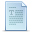Index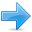Next:Dynamic memory